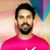# Discussion on: Frequentist and Bayesian coin flipping in JuliaVery nice article. The difference is in terms of interpretation, but can be meaningful in the way you model stuff. The frequentist is assuming that you want to calculate the probability of observing a sample `x` given that the real paramater of the model is theta.
Hence, it makes the assumption that theta is deterministic, and hence, making probabilistic statement regarding theta are non-sense.
The Baysian always presumes a distribution for the parameter.
The two can be somewhat reconciled via what one would call a hierarchical model. Hence, the frequentist would also assume theta to be random, and another parameter (gamma) to be the deterministic one. This is similar to the Bayesian modeling.

The main weakness I see in the Bayesian interpretation comes from the "prior as a degree of belief", which is just bs to me. The weakness in frequentism is assume that parameters are deterministic, when in reality they mostly are not.